## The What of the What?¶

The Chromatic Number of the Plane, or Hadwiger-Nelson problem is a math problem originating in the 1950s, and one which remains unsolved. It came to my attention after a breakthrough by Aubrey D.N.J. de Grey in 2018, and was subsequently the subject of Polymath Project 16 - a collaborative effort from mathematicians from all over the world to improve upon this. I'll do my best to explain what all this means...

First, the problem itself. The Chromatic Number of the Plane is the minimum number of colours it takes to colour an infinite flat surface such that there are no two points which are unit length apart have the same colour. A bit slower that means...

1. Pick a 'unit' length, imagine a toothpick.
2. Pick a number of colours - i.e. 1, 2, 3, 4, 5...
3. Colour an infinite flat surface with an infinite pattern using that number of colours (think: checkerboard, stripes, dots, anything). No part can remain 'uncoloured'.
4. Check it satisfies the condition. You shouldn't be able to place the toothpick anywhere where both ends of it are on the same colour.

If the chromatic number of the plane were 6 (we don't know yet), then there would be a way to colour the plane in 6 colours where you 'couldn't place the toothpick', and there wouldn't be any such colouring in 5 colours. We're not even trying to find what that colouring would be... Yet.

Finding if a specific colour pattern doesn't work is as simple as finding two points which are unit-length apart and are the same colour. You can try come up with some patterns for 2 colours and check if they work - checkerboard, stripes, dots... It's not hard to find a place to put the imaginary toothpick such that both ends lie on the same colour.

Finding an upper bound simply requires finding a colouring for a given number of colours. Here's the best we have - a pattern which works for 7 colours.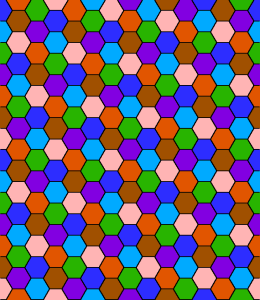Figure 1: A valid colouring of the plane in 7 colours, given the unit length is between the width of one hexagon and the closest distance between two hexagons of the same colour.

Proving that there is no pattern for a given number of colours is going to require a different technique however, but first we'll need some code.

This post is, infact, a jupyter notebook. Feel free to download it from here and the pip install the associated code library I wrote from here run/ tinker with it yourself. The proofs are all generated within. This post is half split between work on the problem itself and algorithmic goodness that can generate and prove properties of graphs faster than anyone else's (maybe). Who knows, maybe you'll come up with some way to solve the problem!

## Starting off simple.¶

We wondered before if the chromatic number of the plane could be as low as 2. A proof it can't be is as simple as drawing a triangle... Why? Well... First lets draw that triangle.

In :
import cnp
from cnp import *
import sympy as sp
from sympy import Symbol, I, S


Define points of an equalateral triangle as points on the complex plane. Note that sympy gives us the exact representation - floating point precision alone isn't enough to determine if points are 'exactly' unit distance apart in some cases.

In :
p0 = S(0)
p1 = S(1)
p2 = S(1)/2 + I*sp.sqrt(1-S(1)/4)
p0, p1, p2

Out:
(0, 1, 1/2 + sqrt(3)*I/2)

Use these points to create a special 'point set', and then convert it to a graph by finding all unit-length edges between the points.

In :
ps = cnp.points.from_complex([p0, p1, p2])
G = cnp.graph.Graph(ps)
G.show(with_labels=True)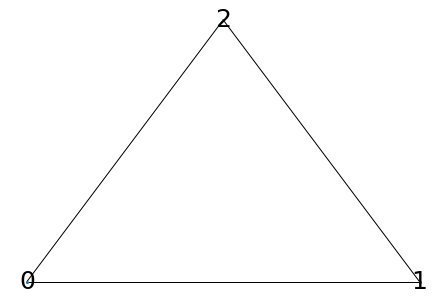We're still considering if it's possible to colour a plane in 2 colours (say, red/green). If such a colouring were to exist, we could place the above unit-length triangle on it. We reduce the infinite colouring of the infinite plane to the 3 points at the vertices - labeled 0, 1, and 2. Each of these points must a colour. Arbitrarally picking 0 is red, we find 1 must be green, 2 red, and then we've 0 and 2 next to one another and the same colour! This is sufficient to prove there's no chromatic colouring of the plane in 2 colours.

The computer can prove this fact just a bit faster though. Behind the scenes it's generating a corresponding boolean satisfiability problem and using a highly optimized solver to check if there is a way to colour this.

In :
for i in range(1,4):
print("Is colourable in", i, "colours?", sat.isColourable(G, i))

Is colourable in 1 colours? False
Is colourable in 2 colours? False
Is colourable in 3 colours? True


## A bit more complicated...¶

We've shown the chromatic number of the plane must be greater than 2, but how about 3? We could generate a bigger graph and see what happens. Lets take the previous triangle, and add it to itself a few times.

In :
p2 = cnp.points.empty()

for i in range(3):
p_rot = cnp.points.rotated(ps, offset=S(0), angle=sp.pi/3*2*i)

H = cnp.graph.Graph(p2)
H.show(with_labels=True)
print("3?", sat.isColourable(H, 3))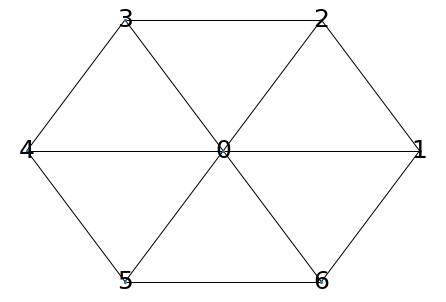3? True


We've made a neat hexagon, but one that's still colourable in 3 colours, unfortunately. Here we rotate about point $1$, by the exact angle required for points $3$ and $5$ to be a unit distance away from their image.

In :
p3 = cnp.points.empty()
for i in range(5):
# Create a copy of H, rotated about (-1,0) slightly.
p_rot = cnp.points.rotated(p2, offset=S(1), angle=i*2*sp.asin(1/sp.sqrt(12)))

H = cnp.graph.Graph(p3)
H.show()
print("3?", sat.isColourable(H, 3))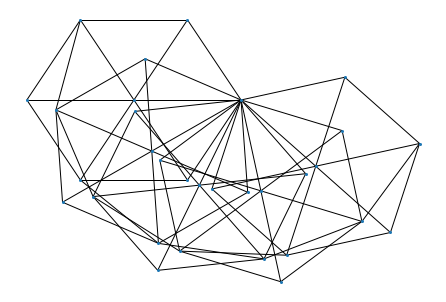3? False


Once again, all edges are unit-length. We have the previous hexagon copied and rotated 5 times. Importantly though, this graph isn't colourable in 3 colours! Or at least, the computer says so. We'd have a better chance of understanding what's going on with fewer edges - maybe we don't need this full graph and could suffice with a subset of vertices.

In :
# Find minimal subgraph that's still not 3 colourable
cnp.sat.optimize(H, 3, extract_MUS=True)
H.show(with_labels=True)

Removing 24 nodes. 7 left.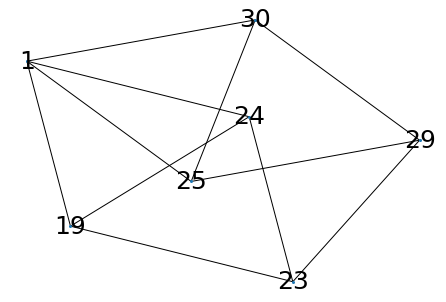Aha! A graph with only 7 vertices which isn't 3-colourable. This graph is special infact, so special it's got it's own wikipedia entry. It's the smallest planar unit graph which is not 3-colourable, and the first graph discovered with such a property.

A quick proof of it's non-3-colourability: Assume it can be coloured with 3. Pick a colour arbitrarally for $1$ (eg. red), then $19$, $24$ must consist of the other two (e.g. blue, green), and with $23$ connected to both, it too must be red. The same logic can be applied along the other 'diamond', showing that $1 == 23 == 29$. Since $23$ and $29$ are unit distance apart, this is a contradiction. The spindle and thus the plane cannot be coloured in 3 colours.

With this, you're up to date with what was known about the problem up until 2018. The CNP was known to be between 4 and 7 (inclusive).

## Getting Big...¶

Aubery De Grey's paper in 2018 opened the floodgates for computer aided optimization and proofs. There has been plenty of very heavy research in chromatic graph theory, yet here we have a breakthrough using not much more than brute force. That's not to take away from the achievement... It's just nice to have a math paper I can actually understand. Similar to before we create a graph, this one's just a bit bigger... To start, a familiar hexagon.

In :
Hp = cnp.points.makeH()
H = graph.Graph(Hp)
H.show()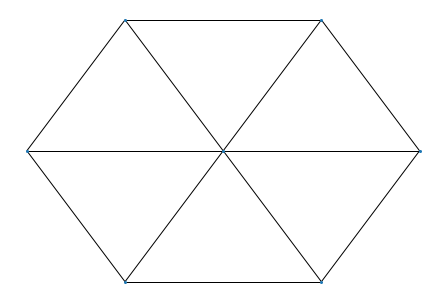We denote this $H$. We're concerned with colourings of $H$ where 3 out of the 6 vertices around the edge are a single colour. This is coined a 'monochromatic triple'. Now take 13 of $H$ and assemble them together.

In :
Jp = cnp.points.makeJ(core=Hp)
J = cnp.graph.Graph(Jp)
J.show(with_labels=True)

Creating: 13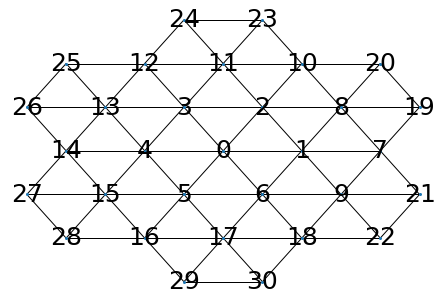Exactly one of the following must hold true for any valid colouring of $J$ (above) in 4 colours:

• The vertices labeled ${0, 7, 10, 12, 14, 16, 18}$ are all the same colour.
• At least one of the subgraph $H$ contains a monochromatic triple. Now, take a copy of $J$, and rotate it about the center so that the images of $7, 10...$ are all unit distant apart from their origional.

This is possible, but not easy, to verify by hand.

In :
Kp = cnp.points.add(Jp,
cnp.points.rotated(Jp, offset=0*I, angle=2*sp.asin(S(1)/4)))
K = cnp.graph.Graph(Kp)
K.show()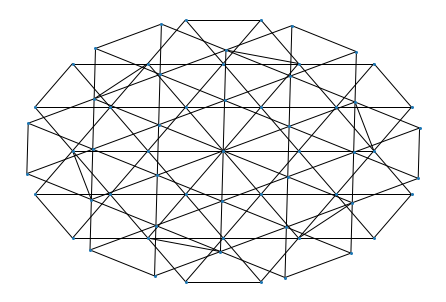Finally, although impossible to see... We have a graph ($J$) that can only be coloured in 4 colours if there is at least one mono-chromatic triple. Now we just somehow construct a $H$ such that it cannot contain a monochromatic triple...

In :
Mp = cnp.points.makeM()
M = cnp.graph.Graph(Mp)
print("Number of vertices:", M.G.number_of_nodes(), "number of edges:", M.G.number_of_edges())
M.show()

# The center hexagon, which makes up the first 7 nodes of M
H = cnp.graph.Graph((Mp[:7], Mp[:7]))
H.show()

31
Number of vertices: 1345 number of edges: 8268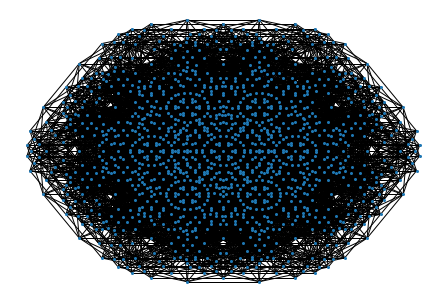The massive $M$ graph, above the $H$ barely visible in the center. We check if this is possible to colour $M$ with $H$ having a monochromatic triple. Here, construct the SAT problem as normal, but add an extra constraint for several of these being the same colour.

In :
%%time

# Create a new constraint, vertices 1, 3, and 5 must never all be the same colour.
tri_nodes = [1, 3, 5]
clauses = cnp.sat.sameColourConstraint(tri_nodes, num_colours=4)

no_constr = cnp.sat.isColourable(M, num_colours=4)
print("Colourable without constraints?", no_constr)
constr = cnp.sat.isColourable(M, num_colours=4, extra_clauses=clauses)
print("Colourable with constraints?   ", constr)

Colourable without constraints? True
Colourable with constraints?    False
CPU times: user 15.2 s, sys: 50.5 ms, total: 15.3 s
Wall time: 15.2 s


Success! We've created a graph that has a hexagon which must not have a monochromatic triple. Now we have both: A $H$ which cannot contain a monochromatic triple, and a graph with lots of copies of $H$, one of which MUST have a monochromatic triple. Maybe you can see where this is going... UHH(Sorry)

In :
%%time
Np = cnp.points.makeN()
N = cnp.graph.Graph(Np)
print("Number of vertices:", N.G.number_of_nodes(), "number of edges:", N.G.number_of_edges())
N.show()

31
Creating: 13
Number of vertices: 20425 number of edges: 151311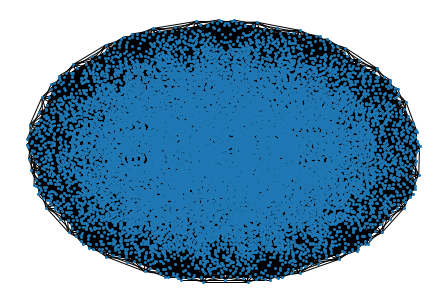CPU times: user 1min 16s, sys: 1.04 s, total: 1min 17s
Wall time: 1min 17s


$N$ is the graph $K$, but with every $H$ replaced with $M$. It's massive. Over 1.5 million edges.

In Aubrey's origional paper, he cleverly proves this monstrosity of a graph is non-4-colourable by proving properties of the subgraphs, as above. His program, in mathematica, takes several minutes to prove that M (with a measily 1345 vertices/ 8268 edges) cannot contain a monochromatic triple...

Unfortunately he didn't know SAT solvers are stupidly fast! Instead of doing that, how about we just plug in the whole thing into a SAT solver and see what we get!?

In :
%%time
cnp.sat.isColourable(N, num_colours=4)

CPU times: user 2min 58s, sys: 292 ms, total: 2min 58s
Wall time: 2min 58s

Out:
False

After several minutes of whirring, we get an answer... False. This graph, and therefore the plane, is not colourable in 4 colours! The chromatic number of the plane must be at least 5!

## Bonus:¶

Why's it so fast? First: Generating the graph. Points are stored in both 'exact' sympy representation and approximate floating point, these can be used to find and remove duplicate points quickly while constructing the graphs. These are found using scipy's cKDTree implementation, which allows for spacial queries in O(Log(n)) time. The same setup is used to find unit-length edges, with candidate matches being created by distance and optionally verified using their exact representations.

Next: Solving it. This project uses pysat, which gives access to lots of SAT solvers. I've found MapleChrono is the fastest for this kind of problem, which isn't too surprising given it won the main track at the 2018 SAT Conference. The SAT problem formulation itself is pretty standard, but there're a few extra tricks for speeding up graph optimization that allows the solver to retain some of the previous graph's solve. All the code's open source anyway... Check it out here!

## Conclusion¶

The chromatic number of the plane must be at least 5. And not more than 7.

I did a lot more work on the CNP problem at the time, like everyone else competing to find the smallest non-chromatic graph, along with finding thin 4-chromatic graphs, subgraphs of larger graphs with interesting properties, among other things. I might be back for a pt 2!